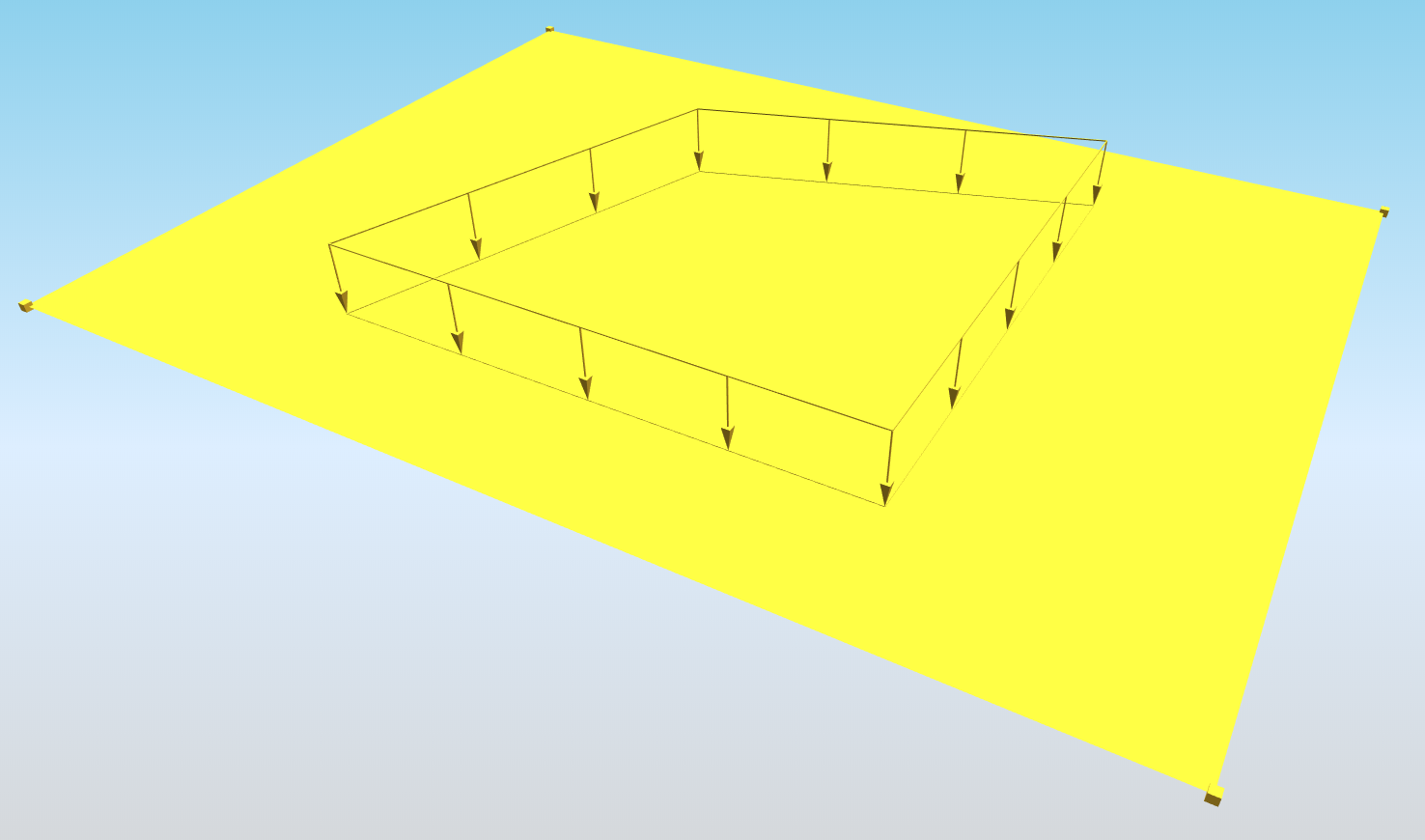# StructuralSurfaceActionFree¶

Warning

object under development

The Free surface load is related to slabs. The load is not defined by the entity it acts on, but by a specific load border. Free loads are defined by means of “loading entities” that may overlap or affect one or more slabs.## Specification in the excel¶

Data type

Example / enum definition

Required

Description

Name

String

FF1

yes

Human readable unique name of the force

Direction

Enum

X

Y

Z

yes

Specifies the base direction of the load

Type

String

Standard

no

This property defines what the load is caused by, E.g. Standard, Wind, Snow, Self weight, Hoar Frost, Predefined, Plane Load, Water Pond, Water Pressure, Soil Pressure, Generated Water, Generated Soil

Distribution

Enum

Uniform

DirectionX

DirectionY

DirectionXY

yes

The load may be either constant across the slab or linearly variable

”DirectionX” and “DirectionY” enums are defining that, the value of the surface load is linearly variable according to the one of the user-selected axis

”DirectionXY” value inclination of surface load is defined in both directions well

q [kN/m2]

String

-10

(for “Distribution” set to “Uniform”)

or

C1:-5; C2:-7

(for “Distribution” set to “Direction*”)

or

C1:-3; C2:-2; C3:-1

(for “Distribution” set to “DirectionXY”)

yes

Specifies the size of the load in kiloNewtons per square meter.

if Distribution is Direction X, Direction Y or Direction XY then the vertexes of the polygon of the free load have to be specified. For distribution Direction X and Direction Y two vertexes, for distribution in Direction XY, three vertexes. The format have to be following:

C”number of vertex of the load polygon” : “value of the load in this vertex”

The number of the vertex corresponds to the coordinate order. So the first coordinate means C1, the second C2 and so on

For further understanding of “C” attributes see notes below table

String

LC5

yes

The name of the load case in StructuralLoadCase to which the force belongs

Validity

Enum

All,
Minus Z,
Minus Z zero,
Z zero,
Plus Z,
Plus Z zero,
From to

yes

All = entities located above as well as below are subject to the defined load
Minus Z = All entities below
Minus Z zero = All entities below and in the plane of the load
Z zero = Only entities in the plane
Plus Z = All entities above
Plus Z zero = All the entities above and in the plane
From to = All entities in the defined range

Validity from [m]

Double

-3.4

yes, if Validity = From to

Validity in the direction of positive Z axis of the load. Load will be generated only above this distance

Validity to [m]

Double

2.1

yes, if Validity = From to

Validity in the direction of positive Z axis of the load. Loads will be generated only below this distance

Local Z direction

Enum

Positive,
Negative

yes

Sets the direction of local Z axis of the load. Local Z axis is always perpendicular to the plane of the load. Positive means that the direction will be in the positive direction of global axis. For more information about the LCS of the load see notes

Coordinate X [m]

Double

0.000; 2.050; 4.850; -2.000

yes

The list of X coordinates of the nodes which define the geometry of the surface free load. Each coordinate is separated by semicolon and space.

Coordinate Y [m]

Double

0.500; 1.050; 2.650; -1.500

yes

The list of Y coordinates of the nodes which define the geometry of the surface free load. Each coordinate is separated by semicolon and space.

Coordinate Z [m]

Double

0.000; 0.000; 0.000; 0.000

yes

The list of Z coordinates of the nodes which define the geometry of the surface free load. Each coordinate is separated by semicolon and space.

Edges

String

Line; Line; Line; Line

yes

Defines shape of the curve between two next nodes. Supported strings are: Line; Bezier; Circle arc; Parabolic arc; Spline. The names are separated by ; (semicolon) and space.

Coordinate system

Enum

Global

Local

Member LCS

yes

Defines co-ordinate system of the member in which the load is applied

Location

Enum

Length

Projection

yes

Specifies whether the load is “put directly on an inclined 2D member” or whether the “projection on plan” is defined.

Id

String

39f238a5-01d0-45cf-a2eb-958170fd4f39

no

Unique attribute designation